Author: Oscar Cronquist Article last updated on December 07, 2020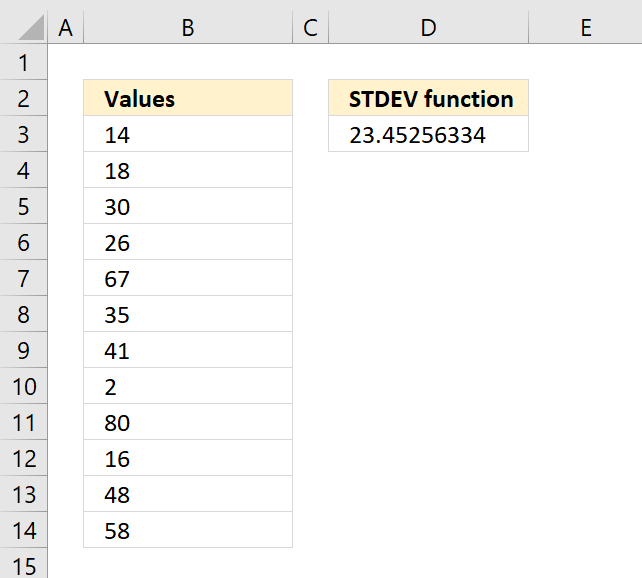The STDEV function calculates the standard deviation of a group of values. The standard deviation shows how much the values differ from the mean value of the group.

Formula in cell C3:

=STDEV(B3:B14)

### Excel Function Syntax

STDEV(number1,[number2],...)

### Arguments

 number1 Required. This argument can be an array of constants or a cell reference to a group of values. [number2] Optional. Up to 254 additional arguments.

### How to use the STDEV function

There are newer better functions that may have improved accuracy, this function is available for compatibility with earlier Excel versions.

### What is Standard Deviation?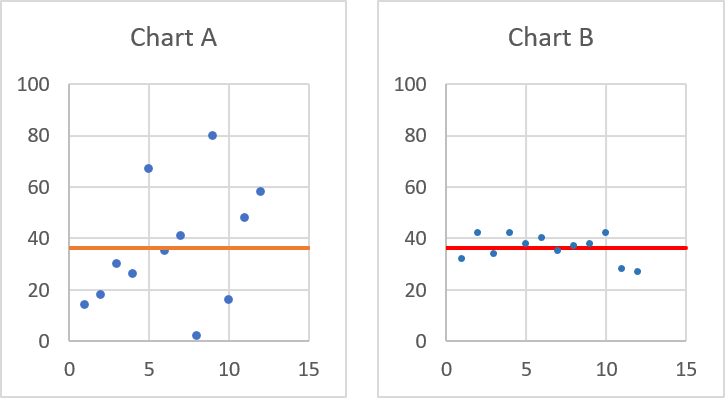Standard deviation tells you how far from the average values are spread out. Both charts above have numbers and an average plotted, they share the same average however, the numbers are not the same.

Chart A above shows that the values are more spread out than the values in chart B. Standard deviation is used in statistics.

### How is the STDEV function calculated?The STDEV function is entered in cell D3, here is how the function calculated the output: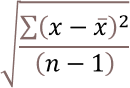x ̅ is the average.
n is how many values.

#### Step 1 - Calculate the average

14 + 18 + 30 + 26 + 67 + 35 + 41 + 2 + 80 + 16 + 48 + 58 = 435

435/12 = 36.25

#### Step 2 - Subtract the average and square the result for all values

(14-36.25)^2 + (18-36.25)^2 + (30-36.25)^2 + (26-36.25)^2 + (67-36.25)^2 + (35-36.25)^2 + (41-36.25)^2 + (2-36.25)^2 + (80-36.25)^2 + (16-36.25)^2 + (48-36.25)^2 + (58-36.25)^2

becomes

(-22.25)^2+(-18.25)^2+(-6.25)^2+(-10.25)^2+(30.75)^2+(-1.25)^2+(4.75)^2+(-34.25)^2+(43.75)^2+(-20.25)^2+(11.75)^2+(21.75)^2

becomes

495.0625+333.0625+39.0625+105.0625+945.5625+1.5625+22.5625+1173.0625+1914.0625+410.0625+138.0625+473.0625

and returns

6050.25

#### Step 3 - Divide with the total count minus 1

6050.25/11 equals 550.022727272727

#### Step 4 - Square root the result

550.022727272727^(1/2) equals 23.4525633411942

### Standard deviation based on a sample

The STDEV function estimates an output based on a sample of values, the STEDV.P however, calcualtes the standard deviation based on the entire population of values.

STDEV function formula:STDEV.P function formula: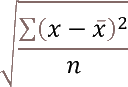These formula differences explain why the functions return different values even if you use the same values.

### What is the difference between the standard deviation and variance?

VAR function formula: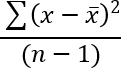STDEV function formula:Standard deviation is the square root of the variance.